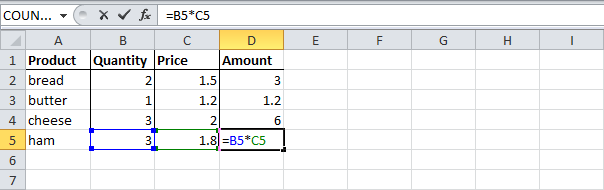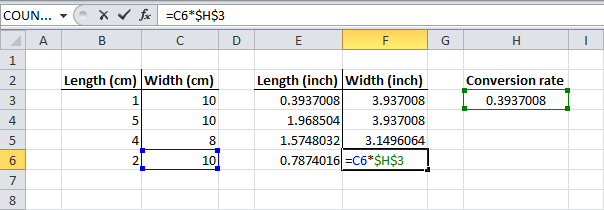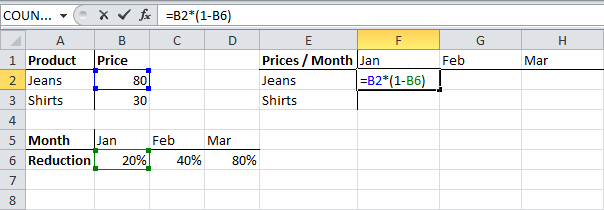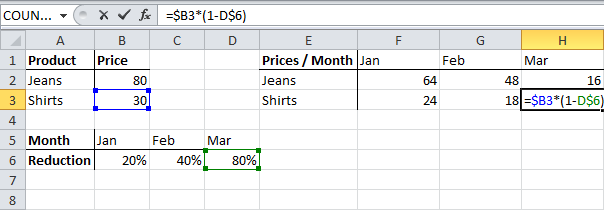# Tech Support Says

For those who seek help in different areas of software and hardware platform.

### Understand and Use "Cell References" in MS ExcelCell references in Excel are very important. Understand the difference between relative, absolute and mixed reference, and you are on your way to success.

## Relative Reference

By default, Excel uses relative reference. See the formula in cell D2 below. Cell D2 references (points to) cell B2 and cell C2. Both references are relative.1. Select cell D2, click on the lower right corner of cell D2 and drag it down to cell D5.Cell D3 references cell B3 and cell C3. Cell D4 references cell B4 and cell C4. Cell D5 references cell B5 and cell C5. In other words: each cell references its two neighbors on the left.

## Absolute Reference

See the formula in cell E3 below.

1. To create an absolute reference to cell H3, place a \$ symbol in front of the column letter and row number of cell H3 (\$H\$3) in the formula of cell E3.2. Now we can quickly drag this formula to the other cells.The reference to cell H3 is fixed (when we drag the formula down and across). As a result, the correct lengths and widths in inches are calculated.

## Mixed Reference

Sometimes we need a combination of relative and absolute reference (mixed reference).

1. See the formula in cell F2 below.2. We want to copy this formula to the other cells quickly. Drag cell F2 across one cell, and look at the formula in cell G2.Do you see what happens? The reference to the price should be a fixed reference to column B. Solution: place a \$ symbol in front of the column letter of cell B2 (\$B2) in the formula of cell F2. In a similar way, when we drag cell F2 down, the reference to the reduction should be a fixed reference to row 6. Solution: place a \$ symbol in front of the row number of cell B6 (B\$6) in the formula of cell F2.

Result:Note: we don't place a \$ symbol in front of the row number of B2 (this way we allow the reference to change from B2 (Jeans) to B3 (Shirts) when we drag the formula down). In a similar way, we don't place a \$ symbol in front of the column letter of B6 (this way we allow the reference to change from B6 (Jan) to C6 (Feb) and D6 (Mar) when we drag the formula across).

3. Now we can quickly drag this formula to the other cells.The references to column B and row 6 are fixed.
Share: